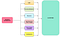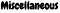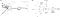# AI & Mathematics

Exploring Mathematical and Statistical Subjects of AI Algorithms.

# Overview:

In this article the above indexes divided in to 2 sections , Section-I (1–4) only theoretical explanations of Maths Subjects and Section-II (5) applied concepts for Neural Network for Multi-class Classification.

# Section — I Theoretical Explanation

Introduction :

AI algorithms based on Mathematics and Statistics, in this article explain importance of Mathematics in AI. Maths behind AI Algorithms is tough to understand and need a steep learning curve. AI algorithms uses Mathematical subjects even though concepts taken from other disciplines (Example: Biological Neuron for Artificial Neural Networks).

Why Mathematics: Below are the few reasons need for Mathematics in AI.

Modules or Fields in AI

There are many modules in AI and I listed few of them according to the book Artificial Intelligence: A Modern Approach by Stuart Russell, Peter Norvig.

Want to know the purpose of the Module in AI below is the diagram describes it, even a newbie can understand the road map of modules.

# AI & its Fields/Modules/Sub-Modules purpose

As outline of AI fields can be categorized in the following diagram.

Mathematical Subjects/Concepts will cover in almost all areas (AI-fields) not only specific to Machine Learning and Deep Learning.

How AI-fields and its required Mathematical subjects/concepts involved in algorithms will be covered in the next article briefly.

# AI-Mathematical subjects and required topics

Going through each subject and mention the major concepts required and where and how to use in AI Algorithms in a short way. By mentioning these reader will be familiar while learning and developing algorithms.

Basic formulas, Functions, Exponential, Logarithms, Euclidean Distance, Plane, Hyperplane, Linear , Non-linear, slope, curves and basics, parabola , circle, etc.,

Introduction: Algebra has multiple variations like Abstract Algebra,Vector Algebra, Linear Algebra.

Abstract Algebra: Laws of Algebra , Groups,homomorphism, Isomorphism, Ring Theory, etc.,

Following are the topics required in Linear Algebra and Vector Algebra. Note that Vector Algebra concepts are few , in some text books they covered in Linear Algebra.

Linear Algebra Concepts: Vectors, Matrices — Types of Matrices(Identity, Inverse,Adjoint) , Tensors, Properties of Matrices (Trace, Determinant, orthogonal,Projections, symmetric, singular ,etc.,), Product Rules- Inner product, Outer product,Vector-Matrix, Matrix Multiplication, Linear Combination of Vectors, Hadamard, Decomposition — Eigen Value Decomposition, SVD, etc., ,Advanced Concepts (uses in QC) — Hilbert Spaces, Tensor product,Hermitian, Unitary, etc.,

You can refresh Linear Algebra in AI & QC, this article will cover almost all topics required in both fields.

Concepts of Vectors applied in ML and Other areas:

Descriptive Statistics: Mean, Variance, Median, Mode, Standard Deviation,Covariance, Expectations, Distributions (Bernoulli, Uniform, Normal (single & multivariate), Poisson, Binomial, Exponential, Gamma), Joint and Marginal Distributions, Probability, axioms of Probability, Conditional Probability, Random Variable,Bayes Rule (Most important) , Chain Rule, Estimation of Parameters: MLE (Maximum Likelihood Estimation), MAP (Maximum A Posterior),Bayesian Networks or Probabilistic Models or Graphical models.

You can see the power of Probability in AI in this article.

Derivatives: Rules of Derivatives: addition, product, division,chain rule, hyperbolic (tanh),applications of derivatives like minima , maxima, etc.,, Integrations (If your using transformations).

Note: We are not using scalar derivatives but these will help in understanding vector and matrix calculus as well as to understand Numerical Computation very well.

Multi variable Calculus, Partial derivatives, Gradient Algorithms.

Variation of Calculus with Linear Algebra: Vector Calculus and Matrix Calculus are most important in Machine Learning and Deep learning

Vector & Matrix Calculus concepts: Gradient , Chain Rule, Jacobians, Hessian.

Following diagram describes Gradient Descent algorithm , it works in Back-propagation (BP) in Neural network architecture for optimizing Parameters.

BP describes Neural Network implementation section.

Concepts: Entropy (Shannon Entropy),Infogain, Cross Entropy, Kullback-Leibler (KL) Divergence. Entropy measures the disorder of the distribution.

Below is the Shannon Entropy diagram describes distributions.

Sets, Sequences,Limits, Metric Spaces, Single-valued and continuous functions, Convergence, Divergence and Taylor-Series.

Extrema, Minima, Maxima, Saddle point, Overflow, Directional derivative, Underflow,Convex,Concave, Convexity, Lagrange’s inequality.

Following concepts used in optimization of weights in ML & DL:

Introduction: Operational Research (OR) is the study of applying Mathematics to business questions. It is a sub-field of Applied Mathematics. OR uses the Mathematics and Statistics to answer optimization question.

Algorithms & Statistics:

OR rely heavily on Algorithms, Mathematics & Statistics. The most important of algorithms in OR are Optimization Algorithms: Algorithms that try to find a maximum or minimum.

Optimization: Challenging is that the best possible solution to a question, given set of constraints. Optimization can be Maximization or Minimization of a cost or benefit.

Mainly we use optimization technique in OR on Cost function.

Sets, Functions, First order Logic, Relations, Data structures,Algorithms,Time & Space Complexity for Algorithms, Recursion, combinatorics,Trees,Graphs, Finite-state Machines, Dynamic Programming,etc.,

Please note that some subjects or concepts be the part of Discrete Mathematics like Probability, Matrices, Boolean Algebra, Languages but these will come in the respective fields.

In the below diagram only well known DM concepts mentioned which are apply in Algorithms. Various other concepts like Finite Automata, Formal Languages, Boolean Algebra, Probability , Matrices are not mentioned due to avoid confusion and collisions.DM Concepts applying in Algorithms for various usagesThere are many subjects/concepts will come into picture and have to learn as and when required

Miscellaneous subjects/concepts: Transformations (Laplace Transformations, Z-Transformations, Fourier- Transformations), distribution functions (Sigmoid, Softmax, Softplus, Tanh,etc.,), Signal Processing, Biological Neuron Concept, Topology, Physics Basics & Control Theory, etc., Only few subjects/concepts mentioned but the list is exhaustible.

# Section-2 Applied Mathematical Concepts in Neural Networks

Let us combine these subjects (mentioned above) in one algorithm and see how these works. For to this , I used Multi Class text Classification example, in this example I use Neural Network architecture and explain how the Maths subjects involved to complete the task.

Following is the Diagram explains how Maths subjects gets involved in Neural Network. Implementing ML algorithm in Neural Networks , so that user can easily understand two learning techniques in one shot.

Neural Network Architecture has many nodes in each layer and we have many layers along with Input and output Layer. In this example I used 1 hidden layer and 1 output layer along with Input layer.

Layers for Multi-class Classification Algorithm:

Input layer : Features or dimensions as Input in the form of Vectors.

Hidden layer : We can have multiple Hidden layers and neurons in each layer. In this example we use only one Hidden Layer.

Output Layer: Soft-max function produces distribution.

Neural Network Architecture build on the concept of neurons. All the Neural Network architectures like NN,CNN,RNN,Generative Models, Auto Encoders, Decoders etc., part of Deep Learning and works on Artificial Neural Networks.

The following diagram comparing Biological Neuron and Artificial Neuron.(a) Biological Neural Network (BNN) & (b) Artificial Neural Network (ANN) and representing in BNN

Artificial Neural Network for Multi-Class Classification.

Neural Network Training can be done in Feedforward Propagation or Forward Propagation and Backward Propagation or Back Propagation.

Every node in each layer is the Element in Vector and every layer is vectored. Feedforward Propagation combining linear combination of weights and inputs (inputs in Input layer and nodes in hidden layer) this can be done using Vector and Matrix product as well as addition of Bias Vector.

Since we have 2 Layers hidden and output layer, so, Feedforward and Back propagation will compute in 2 phases.

## Phase-1 Feedforward

Let us define intermediate variables in above Neural network.

Know the dimensions Of Parameters:

Let’s calculate the intermediate variables in Phase-1.

## Phase-2 Feedforward:

Let’s calculate the intermediate variables in Phase-2. Now Input is hidden layer to the output layer.

After Completion of Feedforward Propagation Back Propagation begins. BP is done in 2 phases. Phase-1 at Output Layer and Phase-2 at Hidden Layer.

Phase-1 Back Propagation:

BP starts from where Feedforward stops. Starting with Cost Function J or H. BP involves many of the Mathematical Subjects such as Real Analysis, Numerical Computation, Convex Optimization, Optimization Algorithms such as Gradient Descent and its variants Algorithms, Matrix Calculus/Vector Calculus,etc.,

Chain Rule and Derivatives of Sigmoid and Softmax:

Intermediate Variables and Back Propagation:

Cost Function for Multi-class Classification

We differentiate Cost Function with respect to parameters in each layer. i.e.,

Starting from the output layer parameters, mathematically it can be described

In the above formula first part’s derivative is

Next Differentiate with respect to Second part in Equation (1)

In the same way, we need to differentiate J with respect to Bias

Phase-2 Back Propagation:

Here I am expanding the chain linked terms and substituted in exact places without giving much explanation, because there are chances to be confused.

Following Diagram clearly mention what Forward and Back Propagation output at each layer.

In simple terms, we train the entire training set , once number of epochs completed or reaching the Minima all parameters will be optimized and gives good results along with accuracy on unknown data.You can see more about Deep Learning usages and how different AI-Fields incorporated in Learning (ML/DL).

Maths and Stats subjects are very important , without this something like a human body without soul.You can treat the mathematical subjects as the pay as you go whenever the requirement comes on the subjects you have to grab and start to work but the above mentioned subjects are minimally required to understand any kind of topic or concept in AI Algorithms.

## References:

Matrix Calculus for Deep Learning: https://arxiv.org/pdf/1802.01528.pdf

Artificial Intelligence: A Modern Approach by Stuart Russell, Peter Norvig.

Written by

Written by

## Shafi

#### Data Architect, Researcher & Guest Faculty in AI ,Autonomous Vehicles & Quantum Computing.